• Solutions• ResourcesBlog

# Faster R-CNN: Down the rabbit hole of modern object detection.css-zjik7{display:-webkit-box;display:-webkit-flex;display:-ms-flexbox;display:flex;}.css-1b6905m{font-family:Open Sans;font-weight:400;font-style:normal;letter-spacing:normal;width:auto;text-align:left;color:#FFFFFF;font-size:16px;line-height:28px;margin:0;display:-webkit-box;display:-webkit-flex;display:-ms-flexbox;display:flex;}.css-1b6905m *{font-size:inherit;}@media (min-width: 600px){.css-1b6905m{font-size:16px;line-height:26px;}}Thu, Jan 18, 2018AuthorsJavier Rey

Share

Previously, we talked about object detection, what it is and how it has been recently tackled using deep learning. If you haven’t read our previous blog post, we suggest you take a look at it before continuing.

In 2017, we decided to get into Faster R-CNN, reading the original paper, and all the referenced papers (and so on and on) until we got a clear understanding of how it works and how to implement it.

We ended up implementing Faster R-CNN in Luminoth, a computer vision toolkit based on TensorFlow which makes it easy to train, monitor and use these types of models. Luminoth has raised an incredible amount of interest and we even talked about it at ODSC Europe, ODSC West and PyImageConf 2018.

Based on all the work developing Luminoth and based on the presentations we did, we thought it would be a good idea to have a blog post with all the details and links we gathered in our research as a future reference for anyone is interested in the topic.

## Background

Faster R-CNN was originally published in NIPS 2015. After publication, it went through a couple of revisions which we'll later discuss. As we mentioned in our previous blog post, Faster R-CNN is the third iteration of the R-CNN papers — which had Ross Girshick as author & co-author.

Everything started with “Rich feature hierarchies for accurate object detection and semantic segmentation” (R-CNN) in 2014, which used an algorithm called Selective Search to propose possible regions of interest and a standard Convolutional Neural Network (CNN) to classify and adjust them. It quickly evolved into Fast R-CNN, published in early 2015, where a technique called Region of Interest Pooling allowed for sharing expensive computations and made the model much faster. Finally came Faster R-CNN, where the first fully differentiable model was proposed.

## Architecture

The architecture of Faster R-CNN is complex because it has several moving parts. We'll start with a high level overview, and then go over the details for each of the components.

It all starts with an image, from which we want to obtain:

• a list of bounding boxes.
• a label assigned to each bounding box.
• a probability for each label and bounding box.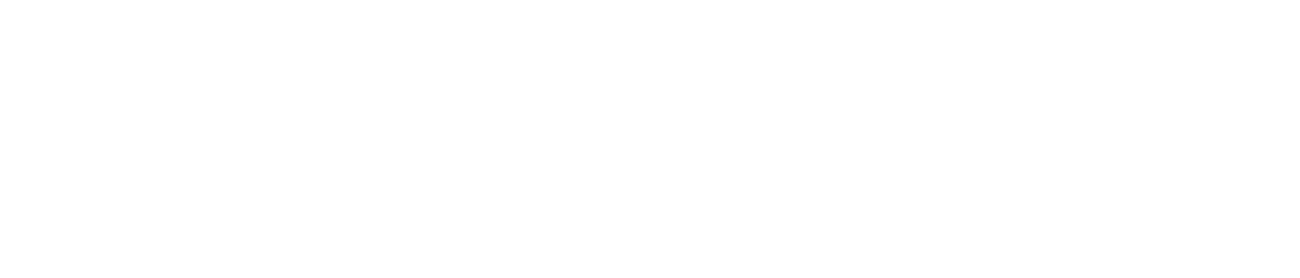Complete Faster R-CNN architecture

The input images are represented as $\mathit{Height} \times \mathit{Width} \times \mathit{Depth}$ tensors (multidimensional arrays), which are passed through a pre-trained CNN up until an intermediate layer, ending up with a convolutional feature map. We use this as a feature extractor for the next part.

This technique is very commonly used in the context of Transfer Learning, especially for training a classifier on a small dataset using the weights of a network trained on a bigger dataset. We'll take a deeper look at this in the following sections.

Next, we have what is called a Region Proposal Network (RPN, for short). Using the features that the CNN computed, it is used to find up to a predefined number of regions (bounding boxes), which may contain objects.

Probably the hardest issue with using Deep Learning (DL) for object detection is generating a variable-length list of bounding boxes. When modeling deep neural networks, the last block is usually a fixed sized tensor output (except when using Recurrent Neural Networks, but that is for another post). For example, in image classification, the output is a $(N,)$ shaped tensor, with $(N,)$ being the number of classes, where each scalar in location $(N,)$ contains the probability of that image being $(N,)$.

The variable-length problem is solved in the RPN by using anchors: fixed sized reference bounding boxes which are placed uniformly throughout the original image. Instead of having to detect where objects are, we model the problem into two parts. For every anchor, we ask:

• Does this anchor contain a relevant object?
• How would we adjust this anchor to better fit the relevant object?

This is probably getting confusing, but fear not, we'll dive into this below.

After having a list of possible relevant objects and their locations in the original image, it becomes a more straightforward problem to solve. Using the features extracted by the CNN and the bounding boxes with relevant objects, we apply Region of Interest (RoI) Pooling and extract those features which would correspond to the relevant objects into a new tensor.

Finally, comes the R-CNN module, which uses that information to:

• Classify the content in the bounding box (or discard it, using “background” as a label).
• Adjust the bounding box coordinates (so it better fits the object).

Obviously, some major bits of information are missing, but that's basically the general idea of how Faster R-CNN works. Next, we'll go over the details on both the architecture and loss/training for each of the components.

## Base network

As we mentioned earlier, the first step is using a CNN pretrained for the task of classification (e.g. using ImageNet) and using the output of an intermediate layer. This may sound really simple for people with a deep learning background, but it's important to understand how and why it works, as well as visualize what the intermediate layer output looks like.

There is no real consensus on which network architecture is best. The original Faster R-CNN used ZF and VGG pretrained on ImageNet but since then there have been lots of different networks with a varying number of weights. For example, MobileNet, a smaller and efficient network architecture optimized for speed, has approximately 3.3M parameters, while ResNet-152 (yes, 152 layers), once the state of the art in the ImageNet classification competition, has around 60M. Most recently, new architectures like DenseNet are both improving results while lowering the number of parameters.

### VGG

Before we talk about which is better or worse, let's try to understand how it all works using the standard VGG-16 as an example.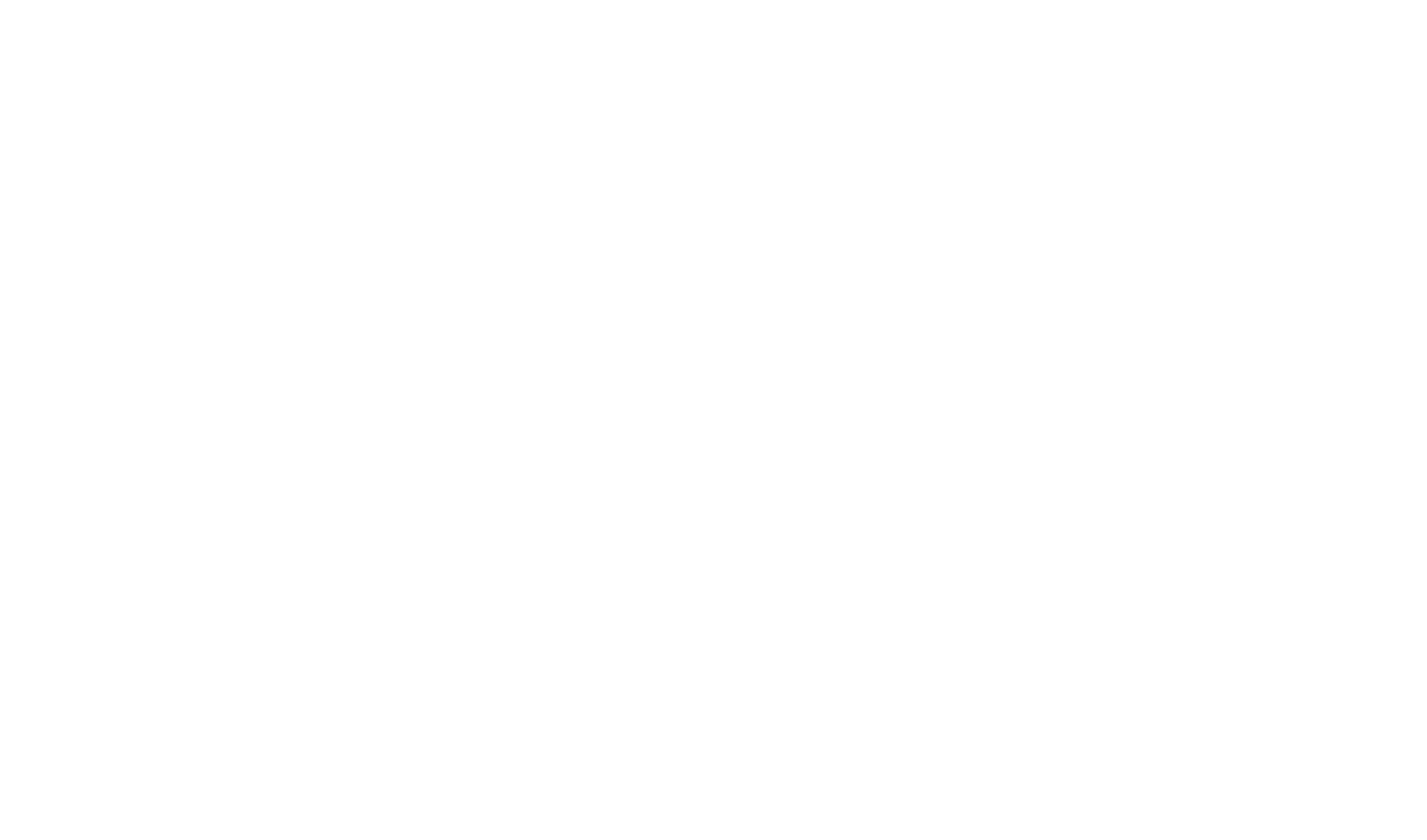VGG architecture

VGG, whose name comes from the team which used it in the ImageNet ILSVRC 2014 competition, was published in the paper “Very Deep Convolutional Networks for Large-Scale Image Recognition” by Karen Simonyan and Andrew Zisserman. By today's standards it would not be considered very deep, but at the time it more than doubled the number of layers commonly used and kickstarted the “deeper → more capacity → better” wave (when training is possible).

When using VGG for classification, the input is a $224 \times 224 \times 3$ tensor (that means a $224 \times 224$ pixel RGB image). This has to remain fixed for classification because the final block of the network uses fully-connected (FC) layers (instead of convolutional), which require a fixed length input. This is usually done by flattening the output of the last convolutional layer, getting a rank 1 tensor, before using the FC layers.

Since we are going to use the output of an intermediate convolutional layer, the size of the input is not our problem. At least, it is not the problem of this module since only convolutional layers are used. Let's get a bit more into low-level details and define which convolutional layer we are going to use. The paper does not specify which layer to use; but in the official implementation you can see they use the output of conv5/conv5_1 layer.

Each convolutional layer creates abstractions based on the previous information. The first layers usually learn edges, the second finds patterns in edges in order to activate for more complex shapes and so forth. Eventually we end up with a convolutional feature map which has spatial dimensions much smaller than the original image, but greater depth. The width and height of the feature map decrease because of the pooling applied between convolutional layers and the depth increases based on the number of filters the convolutional layer learns.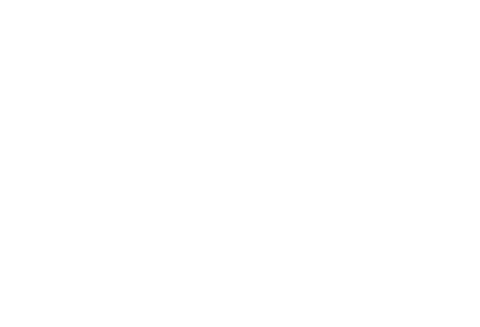Image to convolutional feature map

In its depth, the convolutional feature map has encoded all the information for the image while maintaining the location of the “things” it has encoded relative to the original image. For example, if there was a red square on the top left of the image and the convolutional layers activate for it, then the information for that red square would still be on the top left of the convolutional feature map.

### VGG vs ResNet

Nowadays, ResNet architectures have mostly replaced VGG as a base network for extracting features. Three of the co-authors of Faster R-CNN (Kaiming He, Shaoqing Ren and Jian Sun) were also co-authors of “Deep Residual Learning for Image Recognition”, the original paper describing ResNets.

The obvious advantage of ResNet over VGG is that it is bigger, hence it has more capacity to actually learn what is needed. This is true for the classification task and should be equally true in the case of object detection.

Also, ResNet makes it easy to train deep models with the use of residual connections and batch normalization, which was not invented when VGG was first released.

## Anchors

Now that we are working with a processed image, we need to find proposals, ie. regions of interest for classification. We previously mentioned that anchors are a way to solve the variable length problem, but we skipped most of the explanation.

Our objective is to find bounding boxes in the image. These have rectangular shape and can come in different sizes and aspect ratios. Imagine we were trying to solve the problem knowing beforehand that there are two objects on the image. The first idea that comes to mind is to train a network that returns 8 values: two $x_{min}, y_{min}, x_{max}, y_{max}$ tuples defining a bounding box for each object. This approach has some fundamental problems. For example, images may have different sizes and aspect ratios, having a good model trained to predict raw coordinates can turn out to be very complicated (if not impossible). Another problem is invalid predictions: when predicting $x_{min}, y_{min}, x_{max}, y_{max}$ and $x_{min}, y_{min}, x_{max}, y_{max}$ we have to somehow enforce that $x_{min}, y_{min}, x_{max}, y_{max}$.

It turns out that there is a simpler approach to predicting bounding boxes by learning to predict offsets from reference boxes. We take a reference box $x_{center}, y_{center}, \mathit{width}, \mathit{height}$ and learn to predict $x_{center}, y_{center}, \mathit{width}, \mathit{height}$, which are usually small values that tweak the reference box to better fit what we want.

Anchors are fixed bounding boxes that are placed throughout the image with different sizes and ratios that are going to be used for reference when first predicting object locations.

Since we are working with a convolutional feature map of size $\mathit{conv}_{width} \times \mathit{conv}_{height} \times \mathit{conv}_{depth}$, we create a set of anchors for each of the points in $\mathit{conv}_{width} \times \mathit{conv}_{height} \times \mathit{conv}_{depth}$. It's important to understand that even though anchors are defined based on the convolutional feature map, the final anchors reference the original image.

Since we only have convolutional and pooling layers, the dimensions of the feature map will be proportional to those of the original image. Mathematically, if the image was $w \times h$, the feature map will end up $w \times h$ where $w \times h$ is called subsampling ratio. If we define one anchor per spatial position of the feature map, the final image will end up with a bunch of anchors separated by $w \times h$ pixels. In the case of VGG, $w \times h$.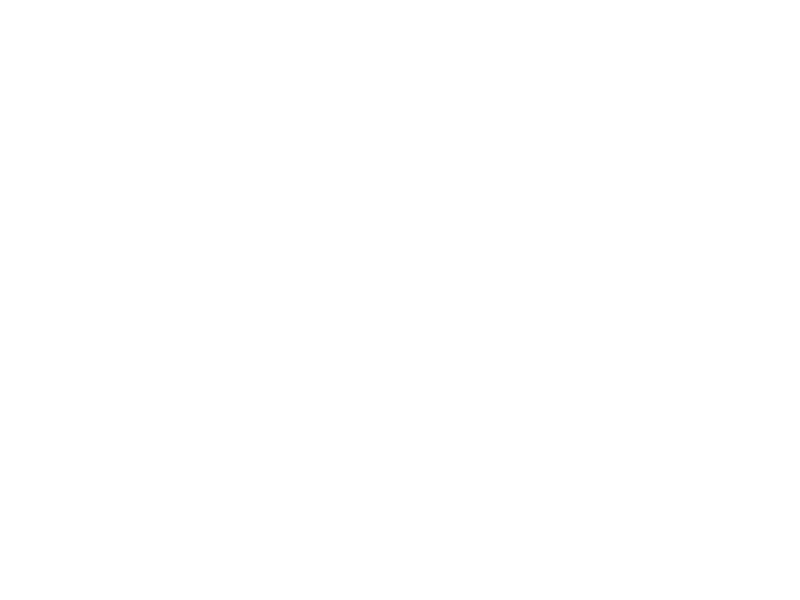Anchor centers throught the original image

In order to choose the set of anchors we usually define a set of sizes (e.g. 64px, 128px, 256px) and a set of ratios between width and height of boxes (e.g. 0.5, 1, 1.5) and use all the possible combinations of sizes and ratios.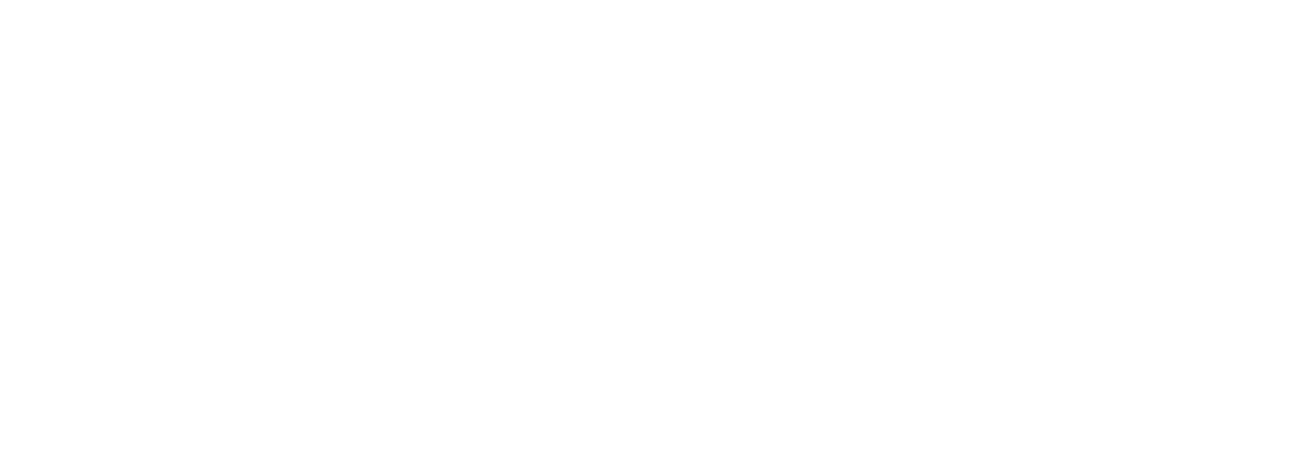Left: Anchors, Center: Anchor for a single point, Right: All anchors

## Region Proposal Network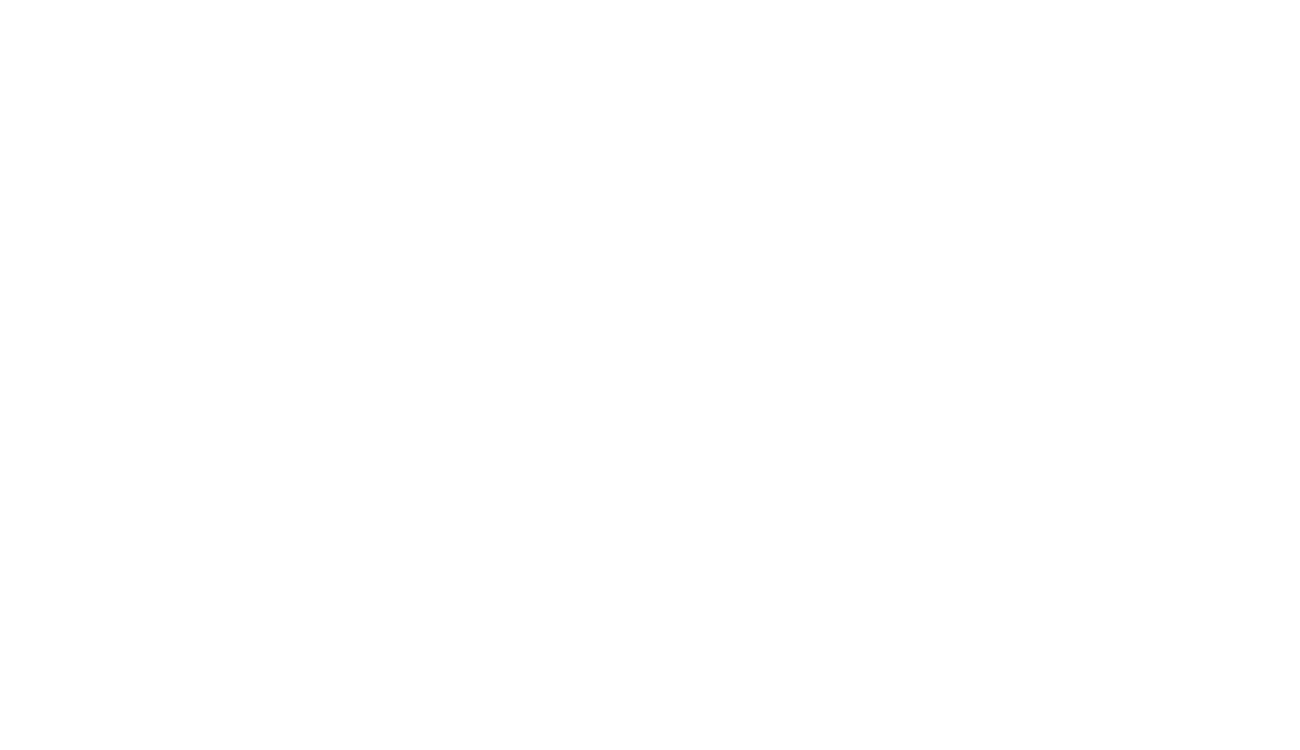The RPN takes the convolutional feature map and generates proposals over the image

As we mentioned before, the RPN takes all the reference boxes (anchors) and outputs a set of good proposals for objects. It does this by having two different outputs for each of the anchors.

The first one is the probability that an anchor is an object. An “objectness score”, if you will. Note that the RPN doesn't care what class of object it is, only that it does in fact look like an object (and not background). We are going to use this objectness score to filter out the bad predictions for the second stage. The second output is the bounding box regression for adjusting the anchors to better fit the object it's predicting.

The RPN is implemented efficiently in a fully convolutional way, using the convolutional feature map returned by the base network as an input. First, we use a convolutional layer with 512 channels and 3x3 kernel size and then we have two parallel convolutional layers using a $1x1$ kernel, whose number of channels depends on the number of anchors per point.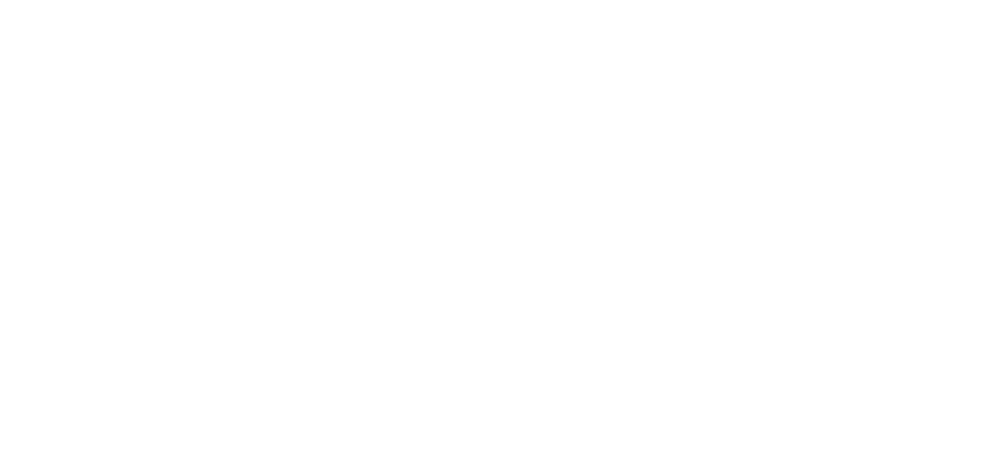Convolutional implementation of an RPN architecture, where k is the number of anchors.

For the classification layer, we output two predictions per anchor: the score of it being background (not an object) and the score of it being foreground (an actual object).

For the regression, or bounding box adjustment layer, we output 4 predictions: the deltas $\Delta_{x_{center}}, \Delta_{y_{center}}, \Delta_{width}, \Delta_{height}$ which we will apply to the anchors to get the final proposals.

Using the final proposal coordinates and their “objectness” score we then have a good set of proposals for objects.

### Training, target and loss functions

The RPN does two different type of predictions: the binary classification and the bounding box regression adjustment.

For training, we take all the anchors and put them into two different categories. Those that overlap a ground-truth object with an Intersection over Union (IoU) bigger than 0.5 are considered “foreground” and those that don't overlap any ground truth object or have less than 0.1 IoU with ground-truth objects are considered “background”.

Then, we randomly sample those anchors to form a mini batch of size 256 — trying to maintain a balanced ratio between foreground and background anchors.

The RPN uses all the anchors selected for the mini batch to calculate the classification loss using binary cross entropy. Then, it uses only those minibatch anchors marked as foreground to calculate the regression loss. For calculating the targets for the regression, we use the foreground anchor and the closest ground truth object and calculate the correct $\Delta$ needed to transform the anchor into the object.

Instead of using a simple L1 or L2 loss for the regression error, the paper suggests using Smooth L1 loss. Smooth L1 is basically L1, but when the L1 error is small enough, defined by a certain $\sigma$, the error is considered almost correct and the loss diminishes at a faster rate.

Using dynamic batches can be challenging for a number of reasons. Even though we try to maintain a balanced ratio between anchors that are considered background and those that are considered foreground, that is not always possible. Depending on the ground truth objects in the image and the size and ratios of the anchors, it is possible to end up with zero foreground anchors. In those cases, we turn to using the anchors with the biggest IoU to the ground truth boxes. This is far from ideal, but practical in the sense that we always have foreground samples and targets to learn from.

### Post processing

Non-maximum suppression Since anchors usually overlap, proposals end up also overlapping over the same object. To solve the issue of duplicate proposals we use a simple algorithmic approach called Non-Maximum Suppression (NMS). NMS takes the list of proposals sorted by score and iterateqs over the sorted list, discarding those proposals that have an IoU larger than some predefined threshold with a proposal that has a higher score.

While this looks simple, it is very important to be cautious with the IoU threshold. Too low and you may end up missing proposals for objects; too high and you could end up with too many proposals for the same object. A value commonly used is 0.6.

Proposal selection After applying NMS, we keep the top N proposals sorted by score. In the paper $N=2000$ is used, but it is possible to lower that number to as little as 50 and still get quite good results.

### Standalone application

The RPN can be used by itself without needing the second stage model. In problems where there is only a single class of objects, the objectness probability can be used as the final class probability. This is because for this case, “foreground” = “single class” and “background” = “not single class”.

Some examples of machine learning problems that can benefit from a standalone usage of the RPN are the popular (but still challenging) face detection and text detection.

One of the advantages of using only the RPN is the gain in speed both in training and prediction. Since the RPN is a very simple network which only uses convolutional layers, the prediction time can be faster than using the classification base network.

## Region of Interest Pooling

After the RPN step, we have a bunch of object proposals with no class assigned to them. Our next problem to solve is how to take these bounding boxes and classify them into our desired categories.

The simplest approach would be to take each proposal, crop it, and then pass it through the pre-trained base network. Then, we can use the extracted features as input for a vanilla image classifier. The main problem is that running the computations for all the 2000 proposals is really inefficient and slow.

Faster R-CNN tries to solve, or at least mitigate, this problem by reusing the existing convolutional feature map. This is done by extracting fixed-sized feature maps for each proposal using region of interest pooling. Fixed size feature maps are needed for the R-CNN in order to classify them into a fixed number of classes.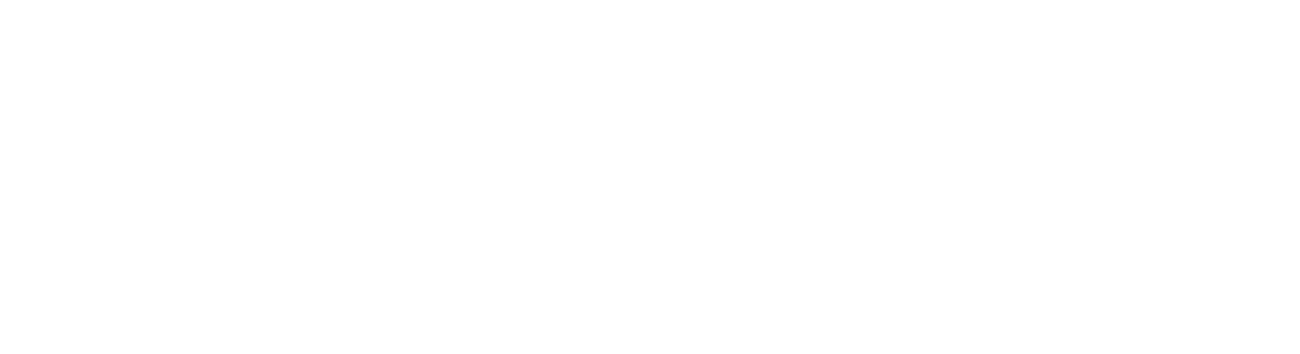Region of Interest Pooling

A simpler method, which is widely used by object detection implementations, including Luminoth's Faster R-CNN, is to crop the convolutional feature map using each proposal and then resize each crop to a fixed sized $14 \times 14 \times \mathit{convdepth}$ using interpolation (usually bilinear). After cropping, max pooling with a 2x2 kernel is used to get a final $14 \times 14 \times \mathit{convdepth}$ feature map for each proposal.

The reason for choosing those exact shapes is related to how it is used next by the next block (R-CNN). It is important to understand that those are customizable depending on the second stage use.

## Region-based Convolutional Neural Network

Region-based convolutional neural network (R-CNN) is the final step in Faster R-CNN's pipeline. After getting a convolutional feature map from the image, using it to get object proposals with the RPN and finally extracting features for each of those proposals (via RoI Pooling), we finally need to use these features for classification. R-CNN tries to mimic the final stages of classification CNNs where a fully-connected layer is used to output a score for each possible object class.

R-CNN has two different goals:

1. Classify proposals into one of the classes, plus a background class (for removing bad proposals).
2. Better adjust the bounding box for the proposal according to the predicted class.

In the original Faster R-CNN paper, the R-CNN takes the feature map for each proposal, flattens it and uses two fully-connected layers of size 4096 with ReLU activation.

Then, it uses two different fully-connected layers for each of the different objects:

• A fully-connected layer with $N+1$ units where $N+1$ is the total number of classes and that extra one is for the background class.
• A fully-connected layer with $4N$ units. We want to have a regression prediction, thus we need $4N$ for each of the N possible classes.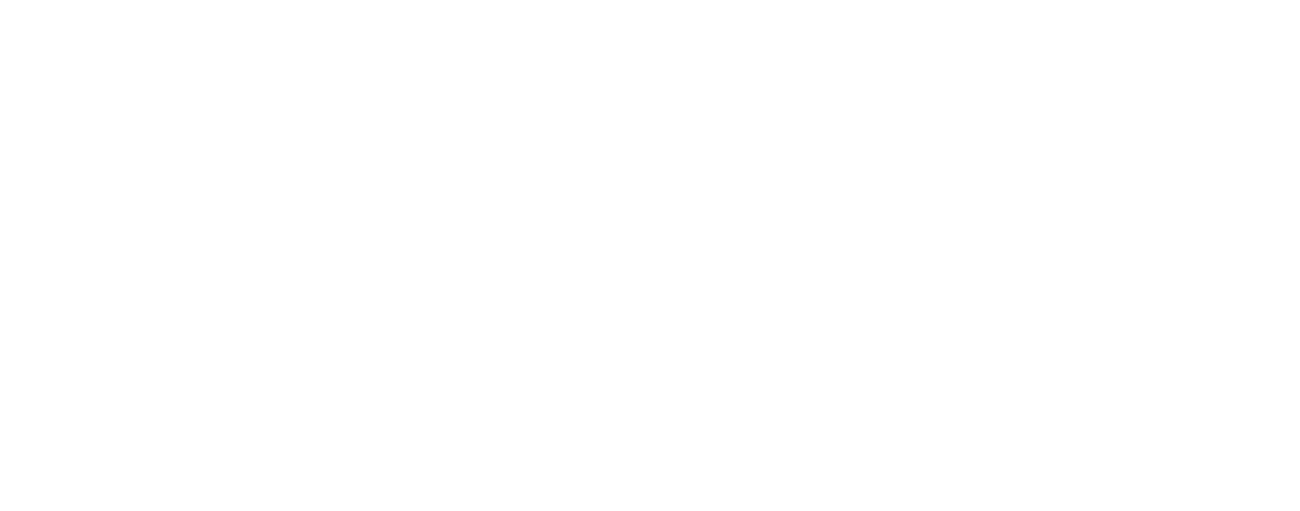R-CNN architecture

### Training and targets

Targets for R-CNN are calculated in almost the same way as the RPN targets, but taking into account the different possible classes. We take the proposals and the ground-truth boxes, and calculate the IoU between them.

Those proposals that have a IoU greater than 0.5 with any ground truth box get assigned to that ground truth. Those that have between 0.1 and 0.5 get labeled as background. Contrary to what we did while assembling targets for the RPN, we ignore proposals without any intersection. This is because at this stage we are assuming that we have good proposals and we are more interested in solving the harder cases. Of course, all these values are hyperparameters that can be tuned to better fit the type of objects that you are trying to find.

The targets for the bounding box regression are calculated as the offset between the proposal and its corresponding ground-truth box, only for those proposals that have been assigned a class based on the IoU threshold.

We randomly sample a balanced mini batch of size 64 in which we have up to 25% foreground proposals (with class) and 75% background.

Following the same path as we did for the RPNs losses, the classification loss is now a multiclass cross entropy loss, using all the selected proposals and the Smooth L1 loss for the 25% proposals that are matched to a ground truth box. We have to be careful when getting that loss since the output of the R-CNN fully connected network for bounding box regressions has one prediction for each of the classes. When calculating the loss, we only have to take into account the one for the correct class.

### Post processing

Similar to the RPN, we end up with a bunch of objects with classes assigned which need further processing before returning them.

In order to apply the bounding box adjustments we have to take into account which is the class with the highest probability for that proposal. We also have to ignore those proposals that have the background class as the one with the highest probability.

After getting the final objects and ignoring those predicted as background, we apply class-based NMS. This is done by grouping the objects by class, sorting them by probability and then applying NMS to each independent group before joining them again.

For our final list of objects, we also can set a probability threshold and a limit on the number of objects for each class.

## Training

In the original paper, Faster R-CNN was trained using a multi-step approach, training parts independently and merging the trained weights before a final full training approach. Since then, it has been found that doing end-to-end, joint training leads to better results.

After putting the complete model together we end up with 4 different losses, two for the RPN and two for R-CNN. We have the trainable layers in RPN and R-CNN, and we also have the base network which we can train (fine-tune) or not.

The decision to train the base network depends on the nature of the objects we want to learn and the computing power available. If we want to detect objects that are similar to those that were on the original dataset on which the base network was trained on, then there is no real need except for trying to squeeze all the possible performance we can get. On the other hand, training the base network can be expensive both in time and on the necessary hardware, to be able to fit the complete gradients.

The four different losses are combined using a weighted sum. This is because we may want to give classification losses more weight relative to regression ones, or maybe give R-CNN losses more power over the RPNs'.

Apart from the regular losses, we also have the regularization losses which we skipped for the sake of brevity but can be defined both in RPN and in R-CNN. We use L2 regularization for some of the layers and depending on which base network being used and if it's trained, it may also have regularization.

We train using Stochastic Gradient Descent with momentum, setting the momentum value to 0.9. You can easily train Faster R-CNN with any other optimizer without bumping into any big problem.

The learning rate starts at $0.001$ and then decreases to $0.001$ after 50K steps. This is one of the hyperparameters that usually matters the most. When training with Luminoth, we usually start with the defaults and tune it from then on.

## Evaluation

The evaluation is done using the standard Mean Average Precision (mAP) at some specific IoU threshold (e.g. mAP@0.5). mAP is a metric that comes from information retrieval, and is commonly used for calculating the error in ranking problems and for evaluating object detection problems.

We won't go into details since these type of metrics deserve a blog post of their own, but the important takeway is that mAP penalizes you when you miss a box that you should have detected, as well as when you detect something that does not exist or detect the same thing multiple times.

## Conclusion

By now, you should have a clear idea of how Faster R-CNN works, why some decisions have been made and some idea on how to be able to tweak it for your specific case. If you want to get a deeper understanding on how it works you should check Luminoth's implementation.

Faster R-CNN is one of the models that proved that it is possible to solve complex computer vision problems with the same principles that showed such amazing results at the start of this new deep learning revolution.

New models are currently being built, not only for object detection, but for semantic segmentation, 3D-object detection, and more, that are based on this original model. Some borrow the RPN, some borrow the R-CNN, others just build on top of both. This is why it is important to fully understand what is under the hood so we are better prepared to tackle future problems.

Solutions